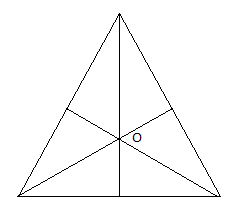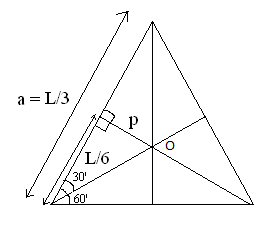#### Thank you for registering.

One of our academic counsellors will contact you within 1 working day.

Click to Chat

1800-5470-145

+91 7353221155

CART 0

• 0
MY CART (5)

Use Coupon: CART20 and get 20% off on all online Study Material

ITEM
DETAILS
MRP
DISCOUNT
FINAL PRICE
Total Price: Rs.

There are no items in this cart.
Continue Shopping

#A rod of mass M kg and length L m is bent in the form of an equilateral triangle as shown. The MI of traingle about a vertical axis perpendicular to the plane of triangle and passing through the centre is?

8 years agoLet a be the side of the equilateral triangle.

Then, L = 3a or a = L/3

thus, M is the mass of the rod the then the three sides of the triangle will be of ''M/3 mass.

and also, by construction, O is the centre or Centroid of the triangle. and Centroid is the points of intersectin of all the medians of the triangle. therefore,

tan30'' = 1/root3 = 6p/l or p = l/6root3 where p is the perpendicular drawn on side AB from point O.

Moment of Inertia of traingle about a vertical axis perpendicular to the plane of triangle and passing through the centre is :

I = [M/3 x L/6root3]^2 x 3 (other three rods of same dimension.]

I = Ml^2/108

is the ansr.

Plz Approve!